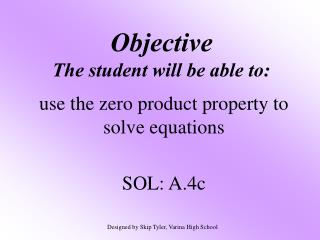Download PresentationObjective The student will be able to:Objective The student will be able to: - PowerPoint PPT Presentation

Download PresentationObjective The student will be able to:
An Image/Link below is provided (as is) to download presentation

Download Policy: Content on the Website is provided to you AS IS for your information and personal use and may not be sold / licensed / shared on other websites without getting consent from its author. While downloading, if for some reason you are not able to download a presentation, the publisher may have deleted the file from their server.

- - - - - - - - - - - - - - - - - - - - - - - - - - - E N D - - - - - - - - - - - - - - - - - - - - - - - - - - -
Presentation Transcript

1. ObjectiveThe student will be able to: use the zero product property to solve equations SOL: A.4c Designed by Skip Tyler, Varina High School

2. Zero Product Property If a • b = 0 then a=0, b=0, or both a and b equal 0.

3. 1. Solve (x + 3)(x - 5) = 0 Using the Zero Product Property, you know that either x + 3 = 0or x - 5 = 0 Solve each equation. x = -3 or x = 5 {-3, 5}

4. 2. Solve (2a + 4)(a + 7) = 0 2a + 4 = 0 or a + 7 = 0 2a = -4 or a = -7 a = -2 or a = -7 {-2, -7}

5. 3. Solve (3t + 5)(t - 3) = 0 3t + 5 = 0 or t - 3 = 0 3t = -5 or t = 3 t = -5/3 or t = 3 {-5/3, 3}

6. Solve (y – 3)(2y + 6) = 0 • {-3, 3} • {-3, 6} • {3, 6} • {3, -6}

7. 4 steps for solving a quadratic equation Set = 0 Factor Split/Solve Check • Set the equation equal to 0. • Factor the equation. • Set each part equal to 0 and solve. • Check your answer on the calculator.

8. 4. Solve x2 - 11x = 0 Set = 0 Factor Split/Solve Check GCF = x x(x - 11) = 0 x = 0 or x - 11 = 0 x = 0 or x = 11 {0, 11}

9. 5. Solve. -24a +144 = -a2 Set = 0 Factor Split/Solve Check Put it in descending order. a2 - 24a + 144 = 0 (a - 12)2 = 0 a - 12 = 0 a = 12 {12}

10. 6. Solve 4m2 + 25 = 20m 4m2 - 20m + 25 = 0 (2m - 5)2 = 0 2m - 5 = 0 2m = 5 m = Set = 0 Factor Split/Solve Check

11. Set = 0 Factor Split/Solve Check 7. Solve x3 + 2x2 = 15x x3 + 2x2 - 15x = 0 x(x2 + 2x - 15) = 0 x(x + 5)(x - 3) = 0 x = 0or x + 5 = 0or x - 3 = 0 {0, -5, 3}

12. Solve a2 – 3a = 40 • {-8, 5} • {-5, 8} • {-8, -5} • {5, 8}

13. Solve 4r3 – 16r = 0 • {-16, 4} • {-4, 16} • {0, 2} • {0, 4} • {-2, 0, 2} The degree will tell you how many answers you have!

14. Maria told this puzzle to her friends. “The product of four times my age and 45 less than three times my age is zero. How old am I?” Find Maria’s age. Let m = Maria’s age. 4m(3m - 45) = 0 4m = 0 or 3m - 45 = 0 m = 0 or 3m = 45 m = 0 or m = 15 0 is not reasonable so Maria is 15 years old!!

15. Find two consecutive integers whose product is 240. Set = 0 Factor Split/Solve Check Let n = 1st integer. Let n + 1 = 2nd integer. n(n + 1) = 240 n2 + n = 240 n2 + n – 240 = 0 (n – 15)(n + 16) = 0

16. (n – 15)(n + 16) = 0 n – 15 = 0 or n + 16 = 0 n = 15 or n = -16 The consecutive integers are 15, 16or -16, -15.